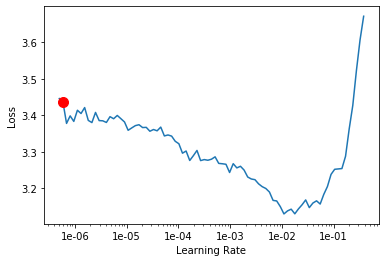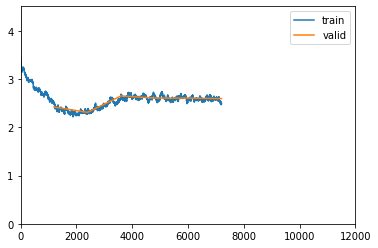# Decoder LSTM loss not improving

I am traning the LSTM decoder for caption generation. My problem is, the loss is not converging as iterations progress instead it reduces in the first epoch then goes up little and remains there.

lr_finder plot:``````learn.freeze() # freezing encoder
learn.fit_one_cycle(10,5e-4,moms=(0.8,0.7))
``````

loss plot:Here is my decoder part with attention network

``````    [Dropout(p=0.5, inplace=False),
Attention(
(enc_att): Linear(in_features=2048, out_features=512, bias=True)
(dec_att): Linear(in_features=512, out_features=512, bias=True)
(att): Linear(in_features=512, out_features=1, bias=True)
(relu): ReLU()
(softmax): Softmax(dim=1)
),
Embedding(4025, 300),
LSTM(2560, 512),
Linear(in_features=2048, out_features=512, bias=True),
Linear(in_features=2048, out_features=512, bias=True),
Linear(in_features=512, out_features=2048, bias=True),
Sigmoid(),
Linear(in_features=512, out_features=4025, bias=True),
Linear(in_features=300, out_features=512, bias=True)]
``````

decoder parameter distribution for different epochs:

I’m struggling to understand what is causing this behavior. Also, looking for some workaround suggestions.

1 Like

Can you provide more detail about the problem and your model? What type of regularization are you using? Have you tried dropping the lr to 1e-6?

@cdparks I resolved it. There was a bug inside the model architecture but forgot to delete this post. Thanks for your response